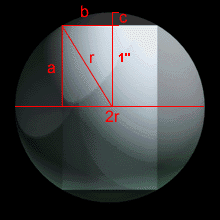# The Billiard BallSkinny must be feeling guilty for having destroyed your cue ball, because this is a bet you can win with ease.

You announce immediately that the volume of the remaining sphere is 4/3 pi cubic inches.

Why? Without thinking you immediately realize that if the problem has a solution (and if it doesn't you win by default, as per the wager), it must work no matter what the radius of the hole was. Including zero! So simply plug in 1 inch into the formula for the volume of a sphere, and there you go.

### And now the hard way...

Of course, we imagine some of you would be curious to see a demonstration of how this would be solved if you didn't know a solution existed. So here we go:

We will call the radius of the original, unmangled sphere, r. We will call the unknown width of the hole "b", the height of the disintegrated spherical cap "c", and half the length of the disintegrated hole "a". The only one of these items whose value we know is "a": one inch. See the diagram to the right and make sure you understand what each refers to.

By the Pythagorean theorem, we know that a2 + b2 = r2. Therefore b= sqrt(r2-1). And c=r-1.

The actual quantity we are looking for is the volume of the remainder of the sphere which equals: (the volume of the sphere) - ((the volume of the cylinder) + 2 (the volume of each cap)).

The formula for the volume of the sphere is 4/3 pi r3. The formula for the volume of the cylinder is (pi * b * 2a). The formula for the volume of the spherical cap is 1/6 pi c ( 3b2 + c2).

We plug in the values we know and get the really pretty formula: (4/3 pi r3) - ((pi * sqrt(r2-1) * 2) + 1/3 pi (r-1) (3 (sqrt(r2-1))2 + (r-1)2))

If we haven't made any errors, all the r's cancel, leaving us with 4/3 pi cubic inches.

3.29 stars. Votes are updated daily.

On a scale of 1 to 5, 1 being among your least favorite, 5 being among your most favorite, how would you rate this puzzle?

1 2 3 4 5